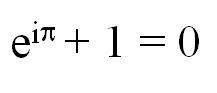## Wednesday, July 29, 2009

### What is pi?

By definition, pi is the ratio of the circumference of a circle to its diameter. Pi is always the same number, no matter which circle you use to compute it. For the sake of usefulness people often need to approximate pi. For many purposes you can use 3.14159, which is really pretty good, but if you want a better approximation you can use a computer to get it. Here's pi to many more digits: 3.14159265358979323846.

A very brief history of pi:
Pi is a very old number. We know that the Egyptians and the Babylonians knew about the existence of the constant ratio pi, although they didn't know its value nearly as well as we do today. They had figured out that it was a little bigger than 3; the Babylonians had an approximation of 3 1/8 (3.125), and the Egyptians had a somewhat worse approximation of 4*(8/9)^2 (about 3.160484), which is slightly less accurate and much harder to work with. The modern symbol for pi was first used in our modern sense in 1706 by William Jones. It(rather than some other Greek letter like Alpha or Omega) was chosen as the letter to represent the number 3.141592... because the letter [pi] in Greek, pronounced like our letter 'p', stands for 'perimeter'.
Pi is an infinite decimal. Unlike numbers such as 3, 9.876, and 4.5, which have finitely many nonzero numbers to the right of the decimal place, pi has infinitely many numbers to the right of the decimal point. If you write pi down in decimal form, the numbers to the right of the 0 never repeat in a pattern. Some infinite decimals do have patterns - for instance, the infinite decimal .3333333... has all 3's to the right of the decimal point, and in the number .123456789123456789123456789... the sequence 123456789 is repeated. However, although many mathematicians have tried to find it, no repeating pattern for pi has been discovered - in fact, in 1768 Johann Lambert proved that there cannot be any such repeating pattern. As a number that cannot be written as a repeating decimal or a finite decimal (you can never get to the end of it) pi is irrational: it cannot be written as a fraction (the ratio of two integers).
Pi shows up in some unexpected places like probability and the 'famous five' equation connecting the five most important numbers in mathematics, 0, 1, e, pi , and i:### NUMBER OF TRAILING ZEROES IN A PRODUCT (PART -2)

Dear all, Please do watch my latest video (dated 10-01-2021) on the topic “NUMBER OF TRAILING ZEROES IN A PRODUCT (PART -2)” VIDEO LINK HERE...